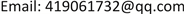1. 引言

2. Super-twisting滑模控制器设计

d x d t = a ( x , t ) + b ( x , t ) u , y = c ( x , t ) (1)

u = K A sgn k 1 y ˙ + k 2 | y | r sgn ( y ) + K B sgn k 3 y ˙ + k 4 | y | r sgn ( y ) (2)

Super-twisting滑模控制是一种二阶滑模控制方案，其控制思想是将开关作用的控制量 u 应用到高阶导数中，这样能有效抑制传统滑模控制中产生的抖振，并能继承传统滑模控制优秀的动态响应和鲁棒性   。本文中将其引入对转速环的控制中。Super-twisting滑模控制律包含了两个部分：一个不连续的滑模变量函数和一个连续导数的函数，分别如式(3)、(4)所示。

u = − K p | y | r sgn ( y ) + u 1 (3)

d u 1 d t = − K I sgn ( y ) (4)

K p > A M B m , K I ≥ 4 A M B m 2 ⋅ B M ( K p + A M ) B m ( K p − A M ) (5)

d 2 y d t 2 = A ( x , t ) + B ( x , t ) u (6)

T e = J d ω d t + D ω + T L (7)

d 2 ω d t 2 = − 1 J ( d T L d t + D d ω d t ) + 1 J d T e d t (8)

T e * = k p | s ω | r sgn ( s ω ) + T e (9)

d T e d t = k i sgn ( s ω ) (10)

3. 改进的直接转矩控制

U k = R k i k + d ψ k d t (11)

ψ ( k ) = ψ ( k − 1 ) + U ( k ) T s (12)

Δ ψ s = | ψ s ( k ) | − | ψ s ( k − 1 ) | ≈ | U ( k ) T s | cos θ υ ψ (13)

Δ ψ s − i n c r e a s e = | V ( k + 1 ) | T s cos θ υ ψ 1 θ υ ψ 1 ∈ ( 30 ˚ , 90 ˚ ) (14)

Δ ψ s − d e c r e a s e = | V ( k + 2 ) | T s cos θ υ ψ 2 θ υ ψ 2 ∈ ( 90 ˚ , 150 ˚ ) (15)

Switching vector table based on 12 section

N = kV kV k + 4V k − 2V k − 6

4. 系统仿真

5. 结论